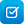#Area of triangles: assessment

TLF ID L8273

Test your understanding of how to use the formula for calculating the area of triangles. Find the area of nine different triangles on a grid. First, predict the area of a chosen triangle on a grid. Find the base and height measurements of the triangle using an animation. Then, substitute the base and height measurements into the formula for calculating the area. Practise applying the formula directly to a range of triangles. Compare the actual area of the triangle with your original estimate and check your answers by viewing your results on a printable report.

Tags: maths

#### Educational details

Key learning objectives
• Students predict the area of triangles.
• Students identify the base and height of triangles.
• Students substitute base and height measurements into the formula for calculating the area of a triangle.
• Students calculate the area of triangles using the area formula.
Educational value
• Assesses students understanding of the formula for calculating the area of triangles.
• Promotes skills in predicting the unknown area of a triangle through the visual stimulation of a grid.
• Enables students to find the base and height measurements of triangles using an animation tool.
• Provides opportunity for students to apply the formula for calculating the area of triangles to different types of triangles.
• Tests students' ability to substitute measurements into a known formula.
• Includes a printable report of the student's answers and the correct values.
• Provides a mechanism for the student and teacher to comment on the student's learning progress.
Year level

7; 8

Learning area
• mathematics
Strand
• Mathematics/Measurement and geometry
Student activity
• Interactives;
• Experiment;
• Analysis;
• Modelling

#### Other details

Contributors
• Subject matter expert
• Name: Jenni Way
• Organisation: University of Western Sydney
• URL: http://www.uws.edu.au/
• Educational validator
• Name: Gayl O’Connor
• Organisation: Education Services Australia
• URL: http://www.esa.edu.au/
• Technical implementer
• Publisher
• Date of contribution: 20 Sep 2013
• Organisation: Education Services Australia
• Address: Melbourne VIC 3000 Australia
• URL: http://www.esa.edu.au/
Access profile
• Colour independence
• Hearing independence
Learning resource type
• Assessment resource
Browsers
• Microsoft Internet Explorer - minimum version: 8.0 (MS-Windows) - maximum version: 9.0 (MS-Windows)
• Firefox - minimum version: (MS-Windows)
• Safari - minimum version: 5.1 (MacOS)
Operating systems
• MacOS - minimum version: 10.6
• MS-Windows - minimum version: XP - maximum version: 7
Rights
• © Education Services Australia Ltd, 2013, except where indicated under Acknowledgements.